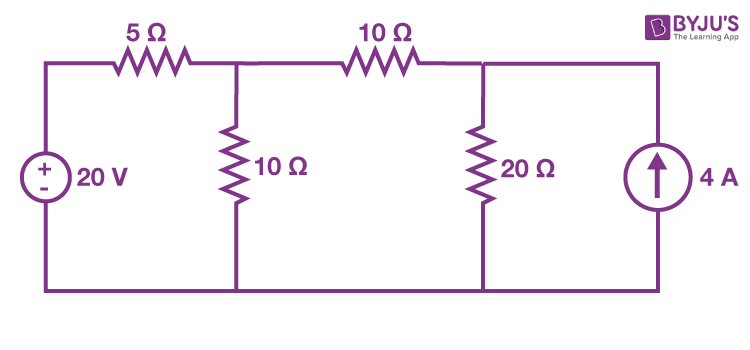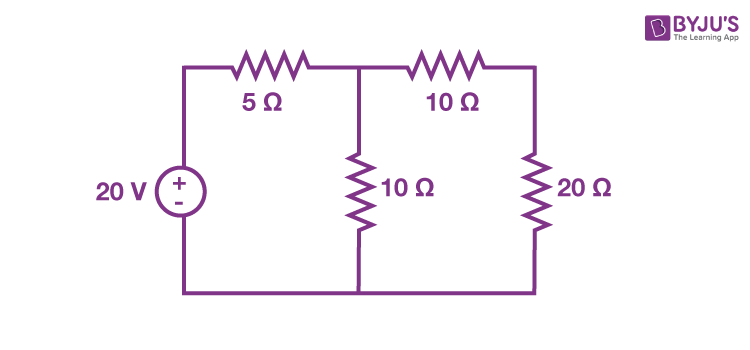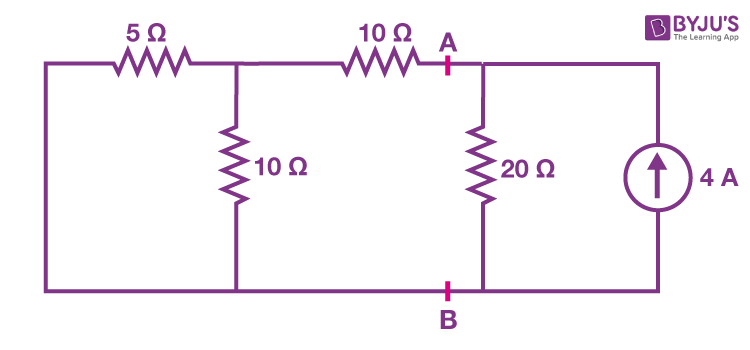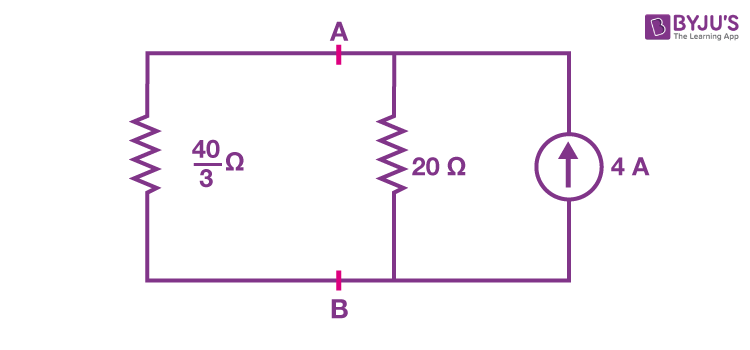Checkout JEE MAINS 2022 Question Paper Analysis : Checkout JEE MAINS 2022 Question Paper Analysis :

# Superposition Theorem

Superposition theorem states that in any linear, bilateral network where more than one source is present, the response across any element in the circuit is the sum of the responses obtained from each source considered separately. In contrast, all other sources are replaced by their internal resistance. Let us know more about the superposition theorem, solved examples and limitations.

## What is Superposition Theorem?

The superposition theorem is a circuit analysis theorem used to solve the network where two or more sources are present and connected.

Superposition theorem states the following:

“If more than one source acts simultaneously in an electric circuit, then the current through any one of the branches of the circuit is the summation of currents which would flow through that branch for each source acting alone, keeping all the other sources dead.”

To calculate the individual contribution of each source in a circuit, the other source must be replaced or removed without affecting the final result. This is done by replacing the voltage source with a short circuit. While removing a voltage source, its value is set to zero. When removing a current source, its value is set to infinite. This is done by replacing the current source with an open circuit.

The superposition theorem is very important in circuit analysis because it converts a complex circuit into a Norton or Thevenin equivalent circuit.

Guidelines to keep in mind while using the superposition theorem

• When you sum the individual contributions of each source, you should be careful while assigning signs to the quantities. It is suggested to assign a reference direction to each unknown quantity. If a contribution from a source has the same direction as the reference direction, it has a positive sign in the sum; if it has the opposite direction, then a negative sign.
• All the components must be linear to use the superposition theorem with circuit currents and voltages.
• It should be noted that the superposition theorem does not apply to power, as power is not a linear quantity.

## How to Apply Superposition Theorem?

• The first step is to select one among the multiple sources present in the bilateral network. Among the various sources in the circuit, any one of the sources can be considered first.
• Except for the selected source, all the sources must be replaced by their internal impedance.
• Using a network simplification approach, evaluate the current flowing through or the voltage drop across a particular element in the network.
• The same considering a single source is repeated for all the other sources in the circuit.
• Upon obtaining the respective response for individual source, perform the summation of all responses to get the overall voltage drop or current through the circuit element.

## Superposition Theorem Solved Example

Let us understand how to use the superposition theorem to analyze circuits with the help of an example.

Example 1: Find the current flowing through 20 Ω using the superposition theorem.Solution:

Step 1: First, let us find the current flowing through a circuit by considering only the 20 V voltage source. The current source can be open-circuited, hence, the modified circuit diagram is shown in the following figure.Step 2: The nodal voltage V1 can be determined using the nodal analysis method.

The nodal equation at node 1 is written as follows:

$$\begin{array}{l}\frac{V_{1}-20}{5}+\frac{V_{1}}{10}+\frac{V_{1}}{10+20}=0\end{array}$$

$$\begin{array}{l}\frac{6V_{1}-120+3V_{1}+V{1}}{30}=0\end{array}$$

$$\begin{array}{l}10V_{1}=120\end{array}$$

$$\begin{array}{l}V_{1}=12\,V\end{array}$$

The current flowing through the 20 Ω resistor can be found using the following equation:

$$\begin{array}{l}I_{1}=\frac{V_{1}}{10+20}\end{array}$$

Substituting the value of the V1 in the above equation, we get

I1 = 0.4 A

Therefore, the current flowing through the 20 Ω resistor to due 20 V voltage source is 0.4 A.

Step 3: Now let us find out the current flowing through the 20 Ω resistor considering only the 4 A current source. We eliminate the 20 V voltage source by short-circuiting it. The modified circuit, therefore, is given as follows:In the above circuit, the resistors 5 Ω and 10 Ω are parallel to each other, and this parallel combination of resistors is in series with the 10 Ω resistor. Therefore, the equivalent resistance will be:

$$\begin{array}{l}R_{AB}=\frac{5\times 10}{5+10}+10=\frac{40}{3}\,\Omega\end{array}$$

Now, the simplified circuit is shown as follows:The current flowing through the 20 Ω resistor can be determined using the current division principle.

$$\begin{array}{l}I_{2}=I_{s}\frac{R_{1}}{R_{1}+R_{2}}\end{array}$$

Substituting the values, we get

$$\begin{array}{l}I_{2}=1.6\,A\end{array}$$

Therefore, the current flowing through the circuit when only 4 A current source is 1.6 A.

Step 4: The summation of currents I1 and I2 will give us the current flowing through the 20 Ω resistor. Mathematically, this is represented as follows:

I = I1 + I2

Substituting the values of I1 and I2 in the above equation, we get

I = 0.4+1.6 = 2 A

Therefore, the current flowing through the resistor is 2 A.

## Limitations of Superposition Theorem

• The theorem does not apply to non-linear circuits. The requisite of linearity indicates that the superposition theorem is only applicable to determine voltage and current but not power. Power dissipation is a nonlinear function that does not algebraically add to an accurate total when only one source is considered at a time.
• The application of the superposition theorem requires two or more sources in the circuit.

## Frequently Asked Questions – FAQs

### What is the superposition theorem?

Superposition theorem is a circuit analysis theorem that is used to solve the network where two or more sources are present and connected.

### Is the superposition theorem valid for AC circuits?

The superposition theorem is valid for AC circuits.

### Is the superposition theorem applicable to power?

The requisite of linearity indicates that the superposition theorem is only applicable to determine voltage and current, but not power. Power dissipation is a nonlinear function that does not algebraically add to an accurate total when only one source is considered at a time.

### Can the superposition theorem be applied to non-linear circuits?

No, the superposition theorem can only be applied to non-linear circuits.

### Why do we use the superposition theorem?

The superposition theorem is very important in circuit analysis because it converts a complex circuit into a Norton or Thevenin equivalent circuit.

## Watch the video and learn about RC circuits and their characteristics.Stay tuned to BYJU’S and Fall in Love with Learning!

Test your Knowledge on Superposition Theorem